## Example Questions

← Previous 1 3

### Example Question #1 : Solution Sets

Find the sum of all of the integer values of x that satisfy the following inequality:

|4 – 2x| < 5

1

10

6

9

4

10

Explanation:

In general, when we take the absolute value of a quantity, we can represent it as either itself, or as its additive inverse.

In other words, |x| = x (if x > 0) or |x| = –x (if x < 0).

Therefore, we can represent |4 – 2x| as either 4 – 2x or as –(4 – 2x). We must consider both of these cases and solve for x. Let’s us first consider the case that |4 – 2x| = 4 – 2x.

4 – 2x < 5

We can add 2x to both sides

4 < 2x + 5

Then subtract 5 from both sides.

–1 < 2x

Divide by 2.

–1/2 < x

x > –0.5

Now, we consider the case that |4 – 2x | = –(4 – 2x).

–(4 – 2x) < 5

Multiply both sides by negative one. Remember, whenever we multiply or divide an inequality by a negative number, we must flip the inequality sign.

4 – 2x > –5

4 > 2x – 5

9 > 2x

Divide by 2,

9/2 > x

4.5 > x

This means that the values of x that satisfy the original quality must be greater than -0.5 AND less than 4.5.

The question asks us to find the sum of the integer values of x that satisfy the inequality. The only integers between -0.5 and 4.5 are the following: 0, 1, 2, 3, and 4.

The sum of 0, 1, 2, 3, and 4 is 10.

### Example Question #1 : How To Find A Solution Set

How many distinct solutions does the following polynomial have?

x(x– 14+ 49) = 0

1

2

3.5

4

3

2

Explanation:

There are 3 solutions: 0, 7, 7.

The correct answer is 2 distinct solutions, however, because 7 occurs twice.  So the two distinct solutions are 0 and 7.

### Example Question #1 : How To Find A Solution Set

Solve x2 – 48 = 0.

x = 0

x = 4√3

x = 4 or x = –4

x = 4√3 or x = –4√3

x = –√48

x = 4√3 or x = –4√3

Explanation:

No common terms cancel out, and this isn't a difference of squares.

Let's move the 48 to the other side: x2 = 48

Now take the square root of both sides: x = √48 or x = –√48. Don't forget the second (negative) solution!

Now √48 = √(3*16) = √(3*42) = 4√3, so the answer is x = 4√3 or x = –4√3.

### Example Question #4 : How To Find A Solution Set

What is the sum of all solutions to the equation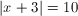?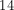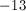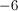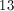Explanation:

If, then either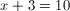or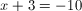.

These two equations yieldandas answers.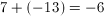### Example Question #1 : How To Find A Solution Set

Using the ordered pairs listed below, which of the following equations is true?

(0, –4)

(2, 0)

(4, 12)

(8, 60)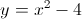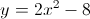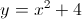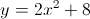Explanation:

You can solve this problem using the guess and check method by substituting the first number in the ordered pair for "x" and the second number for "y". Therfore the correct answer is–4 = 0 – 4

0 = 4 – 4

12 = 16 – 4

60 = 64 – 4

### Example Question #2 : How To Find A Solution Set

Solve for x: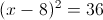x = –2 or –14

x = 2 or –14

x = 14 or –2

x = 14

x = 14 or 2

x = 14 or 2

Explanation:

First, take the square root of both sides: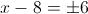Therefore,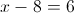or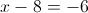Add 8 to both sides of the equation; therefore,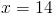or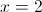### Example Question #1 : How To Find A Solution Set

What is a possible solution to this equation: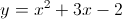?

There is no solution.

(2, 6)

(2, 10)

(3, 18)

(3, 16)

(3, 16)

Explanation:

This equation can be solved using the guess and check method.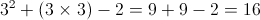Therefore, the ordered pair (3, 16) is the correct answer.

### Example Question #1 : Solution Sets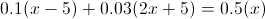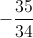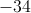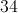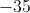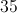Explanation:

First multiply each decimal number in each term by 100 to remove the decimals (to get a whole number you have to multiply 0.03 by 100 to get 3).  You need to do this for terms on both sides of the equal sign.

The second method would be to look for the number of digits to the right of the decimal point (e.g., 0.03 has two digits).  So in this method shift the decimal point to the right two places.

Now the equation looks as follows: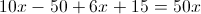Now solve forandwill be equal to.

### Example Question #9 : How To Find A Solution Set

Solve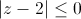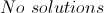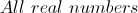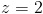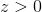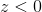Explanation:

Absolute value is the distance from the point to the origin, so the value itself is always poisitive.  The only solution that makes sense for this problem is when the absolute value is equal to zero, or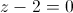and that happens when.

### Example Question #10 : How To Find A Solution Set

In this question we describe the solution set in the form of a line diagram.  Remember a solution to an inequality can be described in the form of (1) Inequality notation, (2) A line diagram, (3) and or an interval notation.

Given a solution set for a linear inequality as shown below: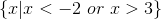what would be the correct representation of the above set in the form of a line diagram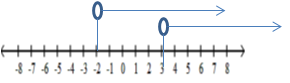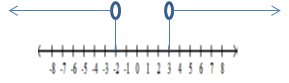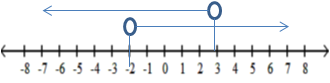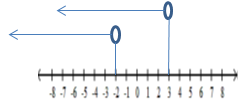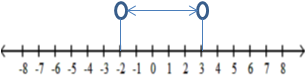The solution lies in the set of real numbers less than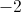or the set of real numbers greater than.